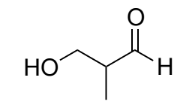# Problem: Write the condensed formula of the following molecule:

###### FREE Expert Solution
82% (255 ratings)
###### Problem Details

Write the condensed formula of the following molecule:Frequently Asked Questions

What scientific concept do you need to know in order to solve this problem?

Our tutors have indicated that to solve this problem you will need to apply the Condensed Structural Formula concept. You can view video lessons to learn Condensed Structural Formula. Or if you need more Condensed Structural Formula practice, you can also practice Condensed Structural Formula practice problems.

What is the difficulty of this problem?

Our tutors rated the difficulty ofWrite the condensed formula of the following molecule: ...as low difficulty.

How long does this problem take to solve?

Our expert Organic tutor, Nicolle took 1 minute and 6 seconds to solve this problem. You can follow their steps in the video explanation above.

What professor is this problem relevant for?

Based on our data, we think this problem is relevant for Professor Ahangari's class at UCF.